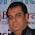Excel Formulas and Functions for Beginners 2021: The Step-by-Step Practical Guide with Examples - KING OF EXCEL

# Excel Formulas and Functions for Beginners 2021: The Step-by-Step Practical Guide with Examples

by James Buffon
• Length: 205 pages
• Edition: 1
• Publisher: Independently published
• Publication Date: 2021-01-28
• Excel’s must-know Formulas and Functions Simplified!

Are you working on a project where you need to perform complex mathematical and financial operations?

Did you know you could use Excel to perform such calculations using the predefined functions and formulae?

Are you looking for a way to learn these functions in a better and simplified way?

If your answer is yes to any of these questions, you have chosen the right book.

Microsoft Excel is an application software that helps you save time and analyze data easily. It has multiple predefined functions you can use to perform complex calculations and analysis and make your job easy. The formulas and functions are the main engines that perform the major operations carried out in Excel. Excel contains over 400 formulas and functions. Regardless of the industry you work in, you must understand the use of these functions so you can improve your analysis and understanding of the data. Use this book as your guide to understand different functions and formulae in Excel.

In this book, you will learn about:

• What Microsoft Excel Is, And Its Individual and Organizational Benefits
• What A Formula and Function Is
• The Formula Tab
• Methods of Entering and Editing of Formulas
• Methods of Entering or Inserting Formulas
• Using the Formula Operators
• The Nested Parentheses
• Working with Functions
• Using the Function Arguments and With No Arguments
• Errors in Formulas and Functions
• Cell Referencing
• Look Up and Reference Functions
• The Vlookup Function
• The Hlookup Function
• The Choose Function
• The Match Function
• The Transpose Function
• The Formulatexts Function
• The Column Function
• The Row Function
• The Index Function
• Logical Functions
• The Math Function
• Statistical Functions
• Date and Time Function
• Text Functions
• The Financial Function
• Some Tips, Tricks and Shortcuts
• … and lots more

To make it easy for you to learn these formulae, there are several screenshots and examples to guide you and make the functions easy for you.

#evba #kingexcel #etipfree #eama

#### 1 comment:

1.A big thank you for sharing this content If anyone looking for best Sas training institute in Delhi Contact Here-+91-9311002620 Or Visit our website https://www.htsindia.com/Courses/business-analytics/sas-training-institute-in-delhi